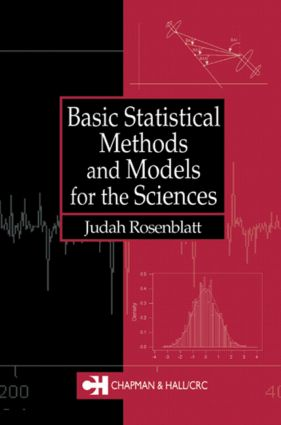# Basic Statistical Methods and Models for the Sciences

## 1st Edition

Chapman and Hall/CRC

296 pages

##### Purchasing Options:\$ = USD
Hardback: 9781584881476
pub: 2001-12-21
\$120.00
x
eBook (VitalSource) : 9781315273754
pub: 2017-07-12
from \$28.98

FREE Standard Shipping!

### Description

The use of statistics in biology, medicine, engineering, and the sciences has grown dramatically in recent years and having a basic background in the subject has become a near necessity for students and researchers in these fields. Although many introductory statistics books already exist, too often their focus leans towards theory and few help readers gain effective experience in using a standard statistical software package.

Designed to be used in a first course for graduate or upper-level undergraduate students, Basic Statistical Methods and Models builds a practical foundation in the use of statistical tools and imparts a clear understanding of their underlying assumptions and limitations. Without getting bogged down in proofs and derivations, thorough discussions help readers understand why the stated methods and results are reasonable. The use of the statistical software Minitab is integrated throughout the book, giving readers valuable experience with computer simulation and problem-solving techniques. The author focuses on applications and the models appropriate to each problem while emphasizing Monte Carlo methods, the Central Limit Theorem, confidence intervals, and power functions.

The text assumes that readers have some degree of maturity in mathematics, but it does not require the use of calculus. This, along with its very clear explanations, generous number of exercises, and demonstrations of the extensive uses of statistics in diverse areas applications make Basic Statistical Methods and Models highly accessible to students in a wide range of disciplines.

### Reviews

"Rosenblatt writes for introductory (non-calculus-based) courses in statistics that offer a clear understanding of statistical procedures together with underlying assumptions and limitations. The author brings a fresh approach to the understanding of statistical concepts by integrating throughout Minitab software, providing valuable insight into computer simulation and problem-solving techniques…Rosenblatt clearly treats the subject matter by carefully wording the explanations and by having readers work with computer-generated data with properties specified by readers. Numerous solved examples; exercises; epilogue with extensions of topics covered. An interesting and useful book. Recommended.

- CHOICE

"This text attempts to address the needs of those who use statistics but are not statisticians. Writing such a text poses two challenges. The first challenge is to present mathematically complex ideas in such a way as to engender an intuitive understanding of the concepts without relying on mathematical detail or rigor. The second is to ground these concepts in application, to show how and why they are important from a practical standpoint…the book is successful on both points…"

- TECHNOMETRICS

INTRODUCTION

Scientific Method

The Aims of Medicine, Science, and Engineering

The Roles of Models and Data

Deterministic and Statistical Models

Probability Theory and Computer Simulation

Definition: Monte Carlo Simulation

CLASSES OF MODELS AND STATISTICAL INFERENCE

Statistical Models - the Frequency Interpretation

Some Useful Statistical Models

Narrowing Down the Class of Potential Models

SAMPLING AND DESCRIPTIVE STATISTICS

Representative and Random Samples

Descriptive Statistics of Location

Descriptive Statistics of Variability

Other Descriptive Statistics

SURVEY OF BASIC PROBABILITY

Introduction

Probability and its Basic Rules

Discrete Uniform Models and Counting

Conditional Probability

Statistical Independence

Systematic Approach to Probability Problems

Random Variables, Expectation and Variance

The Central Limit Theorem and its Applications

INTRODUCTION TO STATISTICAL ESTIMATION

Methods of Estimation

Distribution of Sample Percentiles

Confidence Limits and Confidence Intervals

Confidence Limits and Interval for Binomial p

Comparing Estimators

The Bootstrap

TESTING HYPOTHESES

Introduction

Some Commonly Used Statistical Tests

Types I and II Errors and (Discriminating) Power

The Simulation Approach to Estimating Power

Some Final Issues and Comments

BASIC REGRESSION AND ANALYSIS OF VARIANCE

Introduction

Simple Linear Regression

Multiple Linear Regression

The Analysis of Variance

EPILOGUE

BIBLIOGRAPHY

SELECTED ANSWERS AND SOLUTIONS

INDEX

### Subject Categories

##### BISAC Subject Codes/Headings:
MAT029000
MATHEMATICS / Probability & Statistics / General